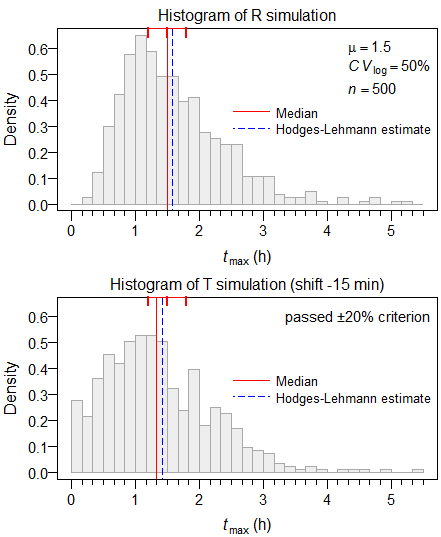## Simulated distributions [BE/BA News]

Hi ElMaestro,

❝ 2. Do we use logs or not?

To get an idea about the simulated distributions, a large sample size and sampling every ten minutes. Shift of T –15 minutes, spread ±30 minutes.Why do we see for T a high density in the first interval? Try this one:

roundClosest <- function(x, y) {   res           <- y * round(x / y)   res[res <= 0] <- y # Force negatives | 0 to the 1st interval   return(res) } HL <- function(x, na.action = na.omit) {   x   <- na.action(x)   y   <- outer(x, x, "+")   res <- median(y[row(y) >= col(y)]) / 2   return(res) } n       <- 500 mu.tmax <- 1.5 # hours CV.tmax <- 0.5 shift   <- -15 # minutes spread  <- 30  # --"-- smpl    <- 10  # --"-- # Sample tmax of R from lognormal distribution # parameterized for the median R       <- rlnorm(n = n, meanlog = log(mu.tmax),                          sdlog = sqrt(log(CV.tmax^2 + 1))) # Introduce shift in location of T shifts  <- runif(n = n, min = shift / 60 - spread / 60, # can be negative!                         max = shift / 60 + spread / 60) T       <- R + shifts # Discretization by the sampling interval 'smpl' R       <- roundClosest(R, smpl / 60) T       <- roundClosest(T, smpl / 60) res     <- data.frame(min    = rep(NA_real_, 3),                       median = NA_real_,                       HL     = NA_real_,                       max    = NA_real_) row.names(res)    <- c("R", "T", "T-R") res[1, c(1:2, 4)] <- quantile(R, probs = c(0, 0.5, 1)) res[1, 3]         <- HL(R) res[2, c(1:2, 4)] <- quantile(T, probs = c(0, 0.5, 1)) res[2, 3]         <- HL(T) res[3, 2:3]       <- res[1, 2:3] - res[2, 2:3] print(round(res, 5))         min  median      HL     max R   0.33333 1.50000 1.58333 7.00000 T   0.16667 1.33333 1.33333 6.66667 T-R      NA 0.16667 0.25000      NA

Since some shifts might be negative, I forced them to the first sampling interval – adding to the ones which are already there. Does that make sense?

Dif-tor heh smusma 🖖🏼 Довге життя Україна!Helmut SchützThe quality of responses received is directly proportional to the quality of the question asked. 🚮
Science QuotesIng. Helmut Schütz